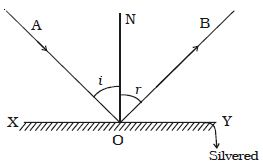# Explain Laws of Reflection

Consider a ray of light, AO, incident on a plane mirror ICY at O. It is reflected along OB. Let the normal ON is drawn at the point of incidence. The angle AON between the incident ray and the normal is called angle of incidence, i (Figure) the angle BON between the reflected ray and the normal is called angle of reflection, r.Experiments show that:

(i) The incident ray, the reflected ray and the normal drawn to the reflecting surface at the point of incidence, all lie in the same plane.

(ii) The angle of incidence is equal to the angle of reflection, i = r.

These are called the laws of reflection.